JAJSE80R October   2017  – November 2021

PRODUCTION DATA

1. 特長
2. アプリケーション
3. 説明
4. Revision History
5. Device Comparison Table
6. Pin Configuration and Functions
7. Specifications
8. Detailed Description
9. Application and Implementation
1. 9.1 Application Information
2. 9.2 Typical Application
1. 9.2.1 TLV900x Low-Side, Current Sensing Application
2. 9.2.2 Single-Supply Photodiode Amplifier
10. 10Power Supply Recommendations
11. 11Layout
12. 12Device and Documentation Support
13. 13Mechanical, Packaging, and Orderable Information

#### パッケージ・オプション

デバイスごとのパッケージ図は、PDF版データシートをご参照ください。

• RUC|14
• D|14
• DYY|14
• PW|14
• RTE|16
• RTE|16
• PW|14
• D|14

#### 9.2.2.2 Detailed Design Procedure

The transfer function between the output voltage (VOUT), the input current, (IIN) and the reference voltage (VREF) is defined in Equation 5.

Equation 5.Where:

Equation 6.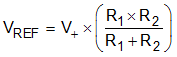Set VREF to 100 mV to meet the minimum output voltage level by setting R1 and R2 to meet the required ratio calculated in Equation 7.

Equation 7.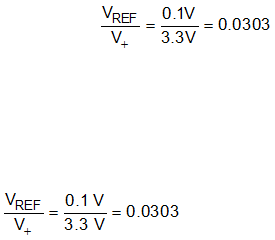The closest resistor ratio to meet this ratio sets R1 to 11.5 kΩ and R2 to 357 Ω.

The required feedback resistance can be calculated based on the input current and desired output voltage.

Equation 8.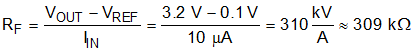Calculate the value for the feedback capacitor based on RF and the desired –3-dB bandwidth, (f–3dB) using Equation 9.

Equation 9.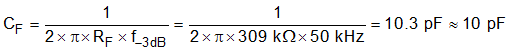The minimum op amp bandwidth required for this application is based on the value of RF, CF, and the capacitance on the INx– pin of the TLV9002 which is equal to the sum of the photodiode shunt capacitance, (CPD) the common-mode input capacitance, (CCM) and the differential input capacitance (CD) as Equation 10 shows.

Equation 10.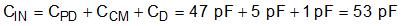The minimum op amp bandwidth is calculated in Equation 11.

Equation 11.The 1-MHz bandwidth of the TLV900x meets the minimum bandwidth requirement and remains stable in this application configuration.